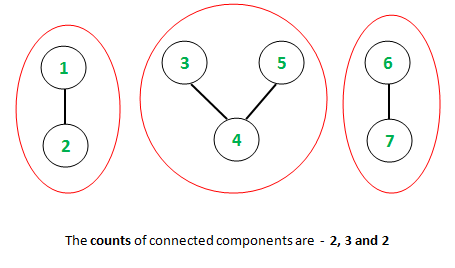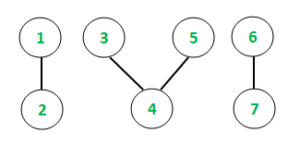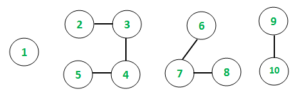# Check if the length of all connected components is a Fibonacci number

Given an undirected graph with V vertices and E edges, the task is to find all the connected components of the graph and check if each of their lengths are a Fibonacci number or not.
For example, consider the following graph.As depicted above, the lengths of the connected components are 2, 3, and 2 which are Fibonacci numbers.
Examples:

Input: E = 4, V = 7Output: Yes
Input: E = 6, V = 10Output: No
Explanation: The lengths of the connected components {1}, {2,3,4,5}, {6,7,8}, {9,10} are 1, 4, 3, 2 respectively.

Approach:
Precompute and store the Fibonacci numbers in a HashSet. Traverse the vertices and generate the Connected components using the DFS approach as explained in this article. Check if all the lengths are present in the precomputed HashSet of Fibonacci numbers.
Below is the implementation of the above approach:

## C++

 `// C++ program to check if the length of ` `// all connected components are a ` `// Fibonacci or not` `#include ` `using` `namespace` `std;`   `// Function to traverse graph using ` `// DFS algorithm and track the` `// connected components` `void` `depthFirst(``int` `v, vector<``int``> graph[],` `                ``vector<``bool``>& visited, ``int``& ans)` `{` `    ``// Mark the current vertex as visited` `    ``visited[v] = ``true``;`   `    ``// Variable ans to keep count of ` `    ``// connected components` `    ``ans++;` `    ``for` `(``auto` `i : graph[v]) {` `        ``if` `(visited[i] == ``false``) {` `            ``depthFirst(i, graph, visited, ans);` `        ``}` `    ``}` `}`   `// Function to check and print if the` `// length of all connected components` `// are a Fibonacci or not` `void` `countConnectedFibonacci(vector<``int``> graph[], ` `                                ``int` `V, ``int` `E)` `{` `    ``// Hash Container (Set) to store ` `    ``// the Fibonacci sequence` `    ``unordered_set<``int``> fibonacci;` `    ``fibonacci.insert(0);` `    ``fibonacci.insert(1);` `    ``// Pre-computation of Fibonacci sequence` `    ``long` `long` `a = 0,b = 1;` `    ``for` `(``int` `i = 2; i < 1001; i++) {` `        ``fibonacci.insert(a + b);` `        ``a = a+b;` `        ``swap(a,b);` `    ``}`   `    ``// Initializing boolean visited array ` `    ``// to mark visited vertices` `    ``vector<``bool``> visited(10001, ``false``);`     `    ``// Following loop invokes DFS algorithm` `    ``for` `(``int` `i = 1; i <= V; i++) {` `        ``if` `(visited[i] == ``false``) {` `            ``// ans variable stores the ` `            ``// length of respective ` `            ``// connected components` `            ``int` `ans = 0;`   `            ``// DFS algorithm` `            ``depthFirst(i, graph, visited, ans);` `            ``if``(fibonacci.find(ans) == fibonacci.end())` `            ``{` `                ``cout << ``"No"``< graph;` `    `  `    ``// Defining the number of edges and vertices` `    ``int` `E = 4,V = 7;`   `    ``// Constructing the undirected graph` `    ``graph.push_back(2);` `    ``graph.push_back(5);` `    ``graph.push_back(4);` `    ``graph.push_back(3);` `    ``graph.push_back(6);` `    ``graph.push_back(3);` `    ``graph.push_back(7);` `    ``graph.push_back(8);` `    `  `    ``countConnectedFibonacci(graph, V, E);` `    ``return` `0;` `}`

## Java

 `// Java program to check if the length of ` `// all connected components are a ` `// Fibonacci or not`   `import` `java.util.*;`   `class` `GFG{`   `// Function to traverse graph using ` `// DFS algorithm and track the` `// connected components` `static` `void` `depthFirst(``int` `v, Vector graph[],` `                ``boolean` `[]visited, ``int` `ans)` `{` `    ``// Mark the current vertex as visited` `    ``visited[v] = ``true``;`   `    ``// Variable ans to keep count of ` `    ``// connected components` `    ``ans++;` `    ``for` `(``int` `i : graph[v]) {` `        ``if` `(visited[i] == ``false``) {` `            ``depthFirst(i, graph, visited, ans);` `        ``}` `    ``}` `}`   `// Function to check and print if the` `// length of all connected components` `// are a Fibonacci or not` `static` `void` `countConnectedFibonacci(Vector graph[], ` `                                ``int` `V, ``int` `E)` `{` `    ``// Hash Container (Set) to store ` `    ``// the Fibonacci sequence` `    ``HashSet fibonacci = ``new` `HashSet();` `    ``fibonacci.add(``0``);` `    ``fibonacci.add(``1``);` `    ``// Pre-computation of Fibonacci sequence` `    ``int` `a = ``0``,b = ``1``;` `    ``for` `(``int` `i = ``2``; i < ``1001``; i++) {` `        ``fibonacci.add(a + b);` `        ``a = a + b;` `        ``a = a + b;` `        ``b = a - b;` `        ``a = a - b;` `    ``}`   `    ``// Initializing boolean visited array ` `    ``// to mark visited vertices` `    ``boolean` `[]visited = ``new` `boolean``[``10001``];`     `    ``// Following loop invokes DFS algorithm` `    ``for` `(``int` `i = ``1``; i <= V; i++) {` `        ``if` `(visited[i] == ``false``) {` `            ``// ans variable stores the ` `            ``// length of respective ` `            ``// connected components` `            ``int` `ans = ``0``;`   `            ``// DFS algorithm` `            ``depthFirst(i, graph, visited, ans);` `            ``if``(!fibonacci.contains(ans))` `            ``{` `                ``System.out.println(``"No"``);` `                ``return``;` `            ``}` `        ``}` `    ``}`   `    ``System.out.println(``"Yes"``);` `}`   `// Driver code` `public` `static` `void` `main(String[] args)` `{` `    ``// Initializing graph in the form of adjacency list` `    ``Vector []graph = ``new` `Vector[``1001``];` `    ``for``(``int` `i = ``0``; i < graph.length; i++)` `        ``graph[i] = ``new` `Vector();` `        `  `    ``// Defining the number of edges and vertices` `    ``int` `E = ``4``,V = ``7``;`   `    ``// Constructing the undirected graph` `    ``graph[``1``].add(``2``);` `    ``graph[``2``].add(``5``);` `    ``graph[``3``].add(``4``);` `    ``graph[``4``].add(``3``);` `    ``graph[``3``].add(``6``);` `    ``graph[``6``].add(``3``);` `    ``graph[``8``].add(``7``);` `    ``graph[``7``].add(``8``);` `    `  `    ``countConnectedFibonacci(graph, V, E);` `}` `}`   `// This code is contributed by 29AjayKumar`

## C#

 `// C# program to check if the length of ` `// all connected components are a ` `// Fibonacci or not` `using` `System;` `using` `System.Collections.Generic;`   `class` `GFG{`   `// Function to traverse graph using ` `// DFS algorithm and track the` `// connected components` `static` `void` `depthFirst(``int` `v, List<``int``> []graph,` `                         ``bool` `[]visited, ``int` `ans)` `{` `    `  `    ``// Mark the current vertex as visited` `    ``visited[v] = ``true``;`   `    ``// Variable ans to keep count of ` `    ``// connected components` `    ``ans++;` `    ``foreach``(``int` `i ``in` `graph[v]) ` `    ``{` `        ``if` `(visited[i] == ``false``)` `        ``{` `            ``depthFirst(i, graph, visited, ans);` `        ``}` `    ``}` `}`   `// Function to check and print if the` `// length of all connected components` `// are a Fibonacci or not` `static` `void` `countConnectedFibonacci(List<``int``> []graph, ` `                                    ``int` `V, ``int` `E)` `{` `    `  `    ``// Hash Container (Set) to store ` `    ``// the Fibonacci sequence` `    ``HashSet<``int``> fibonacci = ``new` `HashSet<``int``>();` `    ``fibonacci.Add(0);` `    ``fibonacci.Add(1);` `    `  `    ``// Pre-computation of Fibonacci sequence` `    ``int` `a = 0,b = 1;` `    ``for``(``int` `i = 2; i < 1001; i++)` `    ``{` `        ``fibonacci.Add(a + b);` `        ``a = a + b;` `        ``a = a + b;` `        ``b = a - b;` `        ``a = a - b;` `    ``}`   `    ``// Initializing bool visited array ` `    ``// to mark visited vertices` `    ``bool` `[]visited = ``new` `bool``;`     `    ``// Following loop invokes DFS algorithm` `    ``for``(``int` `i = 1; i <= V; i++)` `    ``{` `        ``if` `(visited[i] == ``false``) ` `        ``{` `            `  `            ``// ans variable stores the ` `            ``// length of respective ` `            ``// connected components` `            ``int` `ans = 0;`   `            ``// DFS algorithm` `            ``depthFirst(i, graph, visited, ans);` `            `  `            ``if``(!fibonacci.Contains(ans))` `            ``{` `                ``Console.WriteLine(``"No"``);` `                ``return``;` `            ``}` `        ``}` `    ``}` `    ``Console.WriteLine(``"Yes"``);` `}`   `// Driver code` `public` `static` `void` `Main(String[] args)` `{` `    `  `    ``// Initializing graph in the ` `    ``// form of adjacency list` `    ``List<``int``> []graph = ``new` `List<``int``>;` `    ``for``(``int` `i = 0; i < graph.Length; i++)` `        ``graph[i] = ``new` `List<``int``>();` `        `  `    ``// Defining the number of edges and vertices` `    ``int` `E = 4,V = 7;`   `    ``// Constructing the undirected graph` `    ``graph.Add(2);` `    ``graph.Add(5);` `    ``graph.Add(4);` `    ``graph.Add(3);` `    ``graph.Add(6);` `    ``graph.Add(3);` `    ``graph.Add(7);` `    ``graph.Add(8);` `    `  `    ``countConnectedFibonacci(graph, V, E);` `}` `}`   `// This code is contributed by amal kumar choubey `

Output:

```Yes

```

Complexity analysis:
The overall complexity of the program is primarily dictated by three factors, namely, the Depth First Search traversal, the identification of elements from the Fibonacci container, and the pre-computation of the Fibonacci sequence. The DFS traversal boasts a time complexity of O(E + V) where E and V are the edges and vertices of the graph. It takes O(1) time complexity to check if a particular length is present in the HashSet or not. The initial pre-computation has a time complexity of O(N) where N is the number up to which the Fibonacci sequence is stored.
Time Complexity: O(N).
Efficient Approach:
This method basically avoids the Fibonacci pre-computation and uses a simple formulation in order to check if the individual lengths are a Fibonacci number or not. The formula to detect if N is a Fibonacci number is to find the values of 5N2 + 4 and 5N2 – 4 and check if either of them is a perfect square or not. The said formulation had been formulated by I Gessel and can be referred to from this link. The rest of the program has a similar approach as above by computing connected components through DFS traversal.
Below is the implementation of the above approach:

## C++

 `// C++ program to check if the length of ` `// all connected components are a ` `// Fibonacci or not` `#include ` `using` `namespace` `std;`   `// Function to traverse graph using ` `// DFS algorithm and track the` `// connected components` `void` `depthFirst(``int` `v, vector<``int``> graph[],` `                ``vector<``bool``>& visited, ``int``& ans)` `{` `    ``// Mark the current vertex as visited` `    ``visited[v] = ``true``;`   `    ``// Variable ans to keep count of ` `    ``// connected components` `    ``ans++;` `    ``for` `(``auto` `i : graph[v]) {` `        ``if` `(visited[i] == ``false``) {` `            ``depthFirst(i, graph, visited, ans);` `        ``}` `    ``}` `}`   `// Function to check and print if the` `// length of all connected components` `// are a Fibonacci or not` `void` `countConnectedFibonacci(vector<``int``> graph[], ` `                                ``int` `V, ``int` `E)` `{`   `    ``// Initializing boolean visited array ` `    ``// to mark visited vertices` `    ``vector<``bool``> visited(10001, ``false``);`     `    ``// Following loop invokes DFS algorithm` `    ``for` `(``int` `i = 1; i <= V; i++) {` `        ``if` `(visited[i] == ``false``) {` `            ``// ans variable stores the ` `            ``// length of respective ` `            ``// connected components` `            ``int` `ans = 0;`   `            ``// DFS algorithm` `            ``depthFirst(i, graph, visited, ans);` `            `  `            ``double` `x1 = ``sqrt``(5*ans*ans + 4);` `            ``int` `x2 = ``sqrt``(5 * ans * ans + 4);` `            `  `            ``double` `y1 = ``sqrt``(5*ans*ans - 4);` `            ``int` `y2 = ``sqrt``(5 * ans * ans - 4);` `            `  `            ``if``(!(x1 - x2) || !(y1 - y2))` `                ``continue``;` `            ``else` `            ``{` `                ``cout << ``"No"``< graph;` `    `  `    ``// Defining the number of edges and vertices` `    ``int` `E = 4,V = 7;`   `    ``// Constructing the undirected graph` `    ``graph.push_back(2);` `    ``graph.push_back(1);` `    ``graph.push_back(5);` `    ``graph.push_back(2);` `    ``graph.push_back(4);` `    ``graph.push_back(3);` `    ``graph.push_back(6);` `    ``graph.push_back(3);` `    ``graph.push_back(7);` `    ``graph.push_back(8);` `    `  `    ``countConnectedFibonacci(graph, V, E);` `    ``return` `0;` `}`

## Java

 `// Java program to check if the length of ` `// all connected components are a ` `// Fibonacci or not` `import` `java.util.*;` `class` `GFG{`   `// Function to traverse graph using ` `// DFS algorithm and track the` `// connected components` `static` `void` `depthFirst(``int` `v, Vector graph[],` `                    ``Vector visited, ``int` `ans)` `{` `    ``// Mark the current vertex as visited` `    ``visited.add(v, ``true``);`   `    ``// Variable ans to keep count of ` `    ``// connected components` `    ``ans++;` `    ``for` `(``int` `i : graph[v]) ` `    ``{` `        ``if` `(visited.get(i) == ``false``)` `        ``{` `            ``depthFirst(i, graph, visited, ans);` `        ``}` `    ``}` `}`   `// Function to check and print if the` `// length of all connected components` `// are a Fibonacci or not` `static` `void` `countConnectedFibonacci(Vector graph[], ` `                                    ``int` `V, ``int` `E)` `{`   `    ``// Initializing boolean visited array ` `    ``// to mark visited vertices` `    ``Vector visited = ``new` `Vector<>(``10001``);` `    ``for``(``int` `i = ``0``; i < ``10001``; i++)` `        ``visited.add(i, ``false``);`   `    ``// Following loop invokes DFS algorithm` `    ``for` `(``int` `i = ``1``; i < V; i++) ` `    ``{` `        ``if` `(visited.get(i) == ``false``)` `        ``{` `            ``// ans variable stores the ` `            ``// length of respective ` `            ``// connected components` `            ``int` `ans = ``0``;`   `            ``// DFS algorithm` `            ``depthFirst(i, graph, visited, ans);` `            `  `            ``double` `x1 = Math.sqrt(``5` `* ans * ans + ``4``);` `            ``int` `x2 = (``int``)Math.sqrt(``5` `* ans * ans + ``4``);` `            `  `            ``double` `y1 = Math.sqrt(``5` `* ans * ans - ``4``);` `            ``int` `y2 = (``int``)Math.sqrt(``5` `* ans * ans - ``4``);` `            `  `            ``if``((x1 - x2) != ``0` `|| (y1 - y2) != ``0``)` `                ``continue``;` `            ``else` `            ``{` `                ``System.out.println(``"No"``);` `                ``return``;` `            ``}` `        ``}` `    ``}` `    ``System.out.println(``"Yes"``);` `}`   `// Driver code` `public` `static` `void` `main(String[] args)` `{` `    ``// Initializing graph in the form of adjacency list` `    ``@SuppressWarnings``(``"unchecked"``)` `    ``Vector []graph = ``new` `Vector[``1001``];` `    ``for``(``int` `i = ``0``; i < ``1001``; i++)` `        ``graph[i] = ``new` `Vector();` `  `  `    ``// Defining the number of edges and vertices` `    ``int` `E = ``4``,V = ``7``;`   `    ``// Constructing the undirected graph` `    ``graph[``1``].add(``2``);` `    ``graph[``2``].add(``1``);` `    ``graph[``2``].add(``5``);` `    ``graph[``5``].add(``2``);` `    ``graph[``3``].add(``4``);` `    ``graph[``4``].add(``3``);` `    ``graph[``3``].add(``6``);` `    ``graph[``6``].add(``3``);` `    ``graph[``8``].add(``7``);` `    ``graph[``7``].add(``8``);` `    `  `    ``countConnectedFibonacci(graph, V, E);` `}` `}`   `// This code is contributed by Rohit_ranjan`

Output:

```Yes

```

Complexity analysis:
Time Complexity: O(V + E)
This method avoids the earlier pre-computation and uses a mathematical formulation to detect if the individual lengths are Fibonacci numbers. Thus, the computation is achieved in constant time O(1) and constant space as it avoids the use of any HashSet to store the Fibonacci numbers. Thus, the overall complexity of the program in this method is dictated solely through the DFS traversal. Hence, the complexity is O(E + V) where E and V are the numbers of edges and vertices of the undirected graph.My Personal Notes arrow_drop_upCheck out this Author's contributed articles.

If you like GeeksforGeeks and would like to contribute, you can also write an article using contribute.geeksforgeeks.org or mail your article to contribute@geeksforgeeks.org. See your article appearing on the GeeksforGeeks main page and help other Geeks.

Please Improve this article if you find anything incorrect by clicking on the "Improve Article" button below.